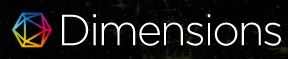### COPRIME GRAPH OF INTEGERS MODULO n GROUP AND ITS SUBGROUPS

Rina Juliana, Masriani Masriani, I Gede Adhitya Wisnu Wardhana, Ni Wayan Switrayni, Irwansyah Irwansyah

DOI: https://doi.org/10.14710/jfma.v3i1.7412

#### Abstract

Coprime Graph is a geometric representation of a group in the form of undirected
graph. The coprime graph of a group G, denoted by \$\Gamma_G\$ is a graph whose vertices are all elements of group G; and two distinct vertices a and b are adjacent if and only if \$(|a|,|b|)=1\$. In this paper, we study coprime graph of integers modulo n group and its subgroups. One of the results is if n is a prime number, then coprime graph of integers modulo n group is a bipartite graph.

PDF

#### References

X.L. Ma, H.Q.Wei, and L.Y. Yang, ”The coprime graph of a group,” International Journal

of Group Theory, vol. 3, no. 3, pp. 13–23, 2014.

H.M. Dorbidi, ”A note on the coprime graph of a group,” International Journal of Group

Theory, vol. 5, no. 4, pp. 17–22, 2016.

R. Munir, Matematika Diskrit. Bandung: Penerbit Informatika, 2010.

### Refbacks

• There are currently no refbacks.

### Publisher:

Department of Mathematics, Faculty of Science and Mathematics, Diponegoro University

Mailing address: Jl. Prof Soedarto, SH, Tembalang, Semarang, Indonesia 50275

Telp./Fax             : (+6224) 70789493 / (+62224) 76480922

Website              : www.math.fsm.undip.ac.id